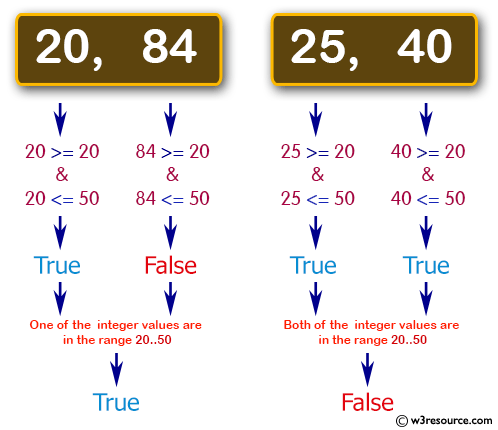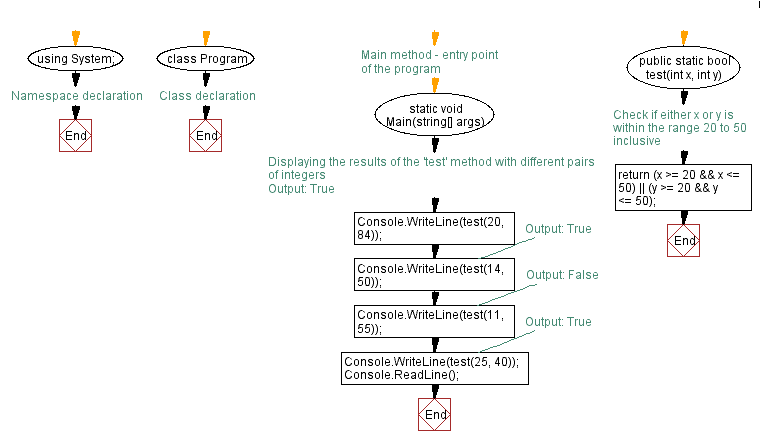﻿ C# - Check whether 2 integer values are in the range 20..50# C# Sharp Basic Algorithm Exercises: Check whether two given integer values are in the range 20..50 inclusive

## C# Sharp Basic Algorithm: Exercise-16 with Solution

Write a C# Sharp program to check whether two given integer values are in the range 20..50 inclusive. Return true if 1 or other is in the said range otherwise false.

Pictorial Presentation:Sample Solution:

C# Sharp Code:

``````using System;

namespace exercises
{
class Program
{
static void Main(string[] args)
{
Console.WriteLine(test(20, 84));
Console.WriteLine(test(14, 50));
Console.WriteLine(test(11, 45));
Console.WriteLine(test(25, 40));
}

public static bool test(int x, int y)
{
return (x <= 20 || y >= 50) || (y <= 20 || x >= 50);
}
}
}
```
```

Sample Output:

```True
True
True
False```

Flowchart:C# Sharp Code Editor:

Improve this sample solution and post your code through Disqus

What is the difficulty level of this exercise?

Test your Programming skills with w3resource's quiz.

﻿